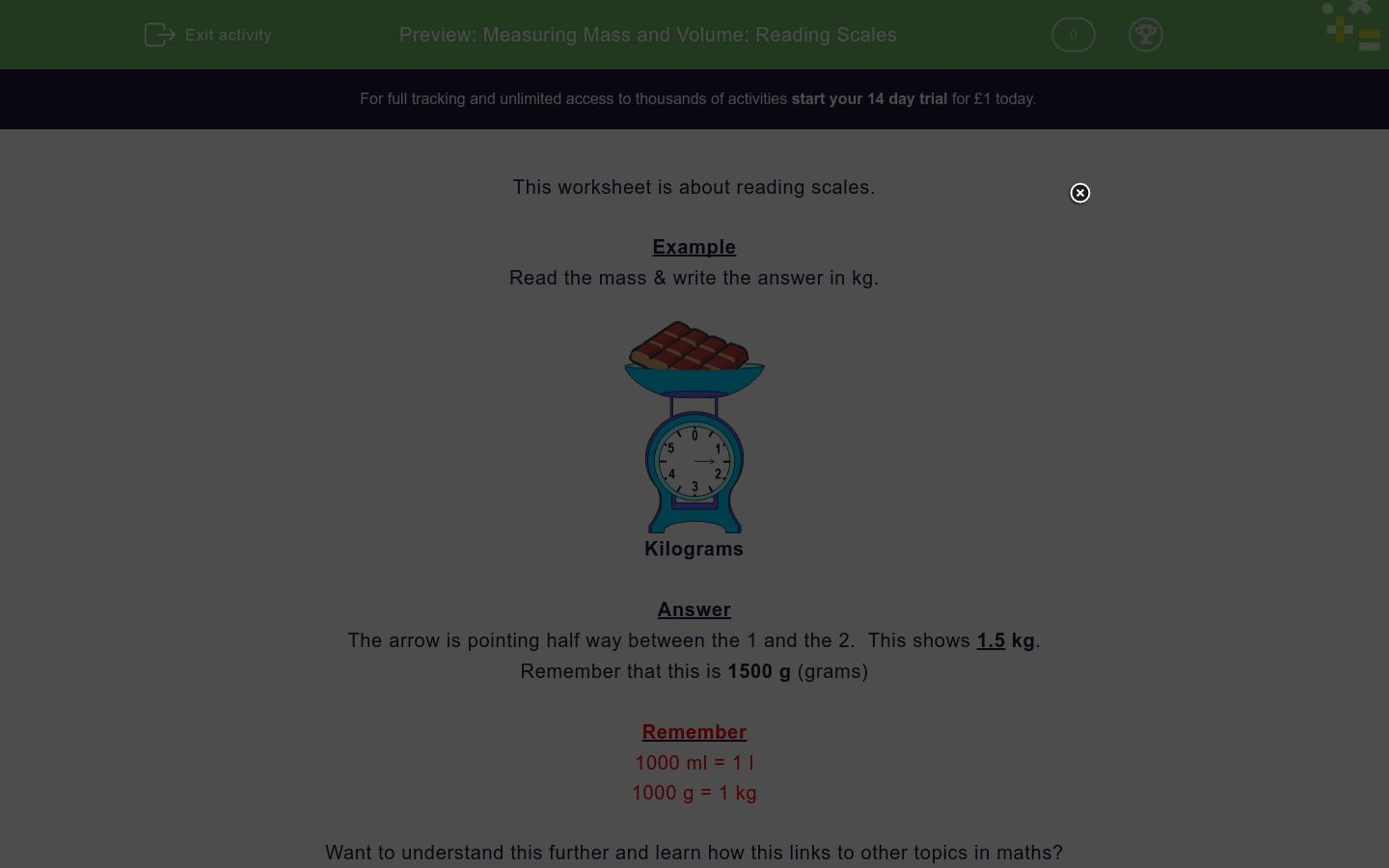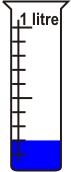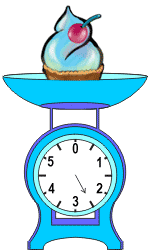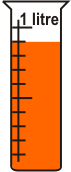# Measuring Mass and Volume: Reading Scales

In this worksheet, students practise measuring weight and volume using a simple set of scales.Key stage:  KS 2

Curriculum topic:   Measurement

Curriculum subtopic:   Measure, Compare, Add/Subtract Lengths, Mass, and Volume/Capacity

Difficulty level:\$appendString = '&new=1'; \$_SESSION['guest_aId'] = rand(); \$urlToRedirect = FRAMEWORK_PATH . 'preview/worksheet/introduction?aId=' . \$_SESSION['guest_aId'] . '&wId=' . \$wsData['id'] . '&usertype=guest' . \$appendString; ?>

### QUESTION 1 of 10

ExampleKilograms

The arrow is pointing half way between the 1 and the 2.  This shows 1.5 kg.

Remember that this is 1500 g (grams)

Remember

1000 ml = 1 l

1000 g = 1 kg

Want to understand this further and learn how this links to other topics in maths?
Why not watch this video?

How much liquid is in this jar? Read the scale & write the answer in ml. (don't include the units)How much liquid is in this jar? Read the scale & write the answer in ml. (don't include the units)Read the mass and write the answer in g. (don't include the units)kilograms

Read the mass and write the answer in kg. (don't include the units)kilograms

Read the mass and write the answer in kilograms. (don't include the units)kilograms

Read the mass and write the answer in kg. (don't include the units)kilograms

How much liquid is there in the jar?

Read the scale & write the answer in ml. (don't include the units)How much liquid is there in the jar?

Read the scale & write the answer in litres. (don't include the units)How much liquid is there in the jar?

Read the scale & write the answer in litres. (don't include the units)• Question 1

How much liquid is in this jar? Read the scale & write the answer in ml. (don't include the units)1000
EDDIE SAYS
1 litre = 1000 ml
• Question 2

How much liquid is in this jar? Read the scale & write the answer in ml. (don't include the units)200
• Question 3

Read the mass and write the answer in g. (don't include the units)kilograms

500
EDDIE SAYS
0.5 kilograms = 500 grams
• Question 4

Read the mass and write the answer in kg. (don't include the units)kilograms

2.5
EDDIE SAYS
• Question 5

Read the mass and write the answer in kilograms. (don't include the units)kilograms

5
EDDIE SAYS
• Question 6

Read the mass and write the answer in kg. (don't include the units)kilograms

0.5
EDDIE SAYS
The scale reads half a kilogram (so, 0.5 kg)
• Question 7

How much liquid is there in the jar?

Read the scale & write the answer in ml. (don't include the units)400
EDDIE SAYS
0.4 litres = 400 ml
• Question 8

How much liquid is there in the jar?

Read the scale & write the answer in litres. (don't include the units)0.6
EDDIE SAYS
The scale reads 0.6, which is 0.6 litres or 600 ml
• Question 9

How much liquid is there in the jar?

Read the scale & write the answer in litres. (don't include the units)0.9
EDDIE SAYS
The scale reads 0.9 litres, or 900 ml
---- OR ----

Sign up for a £1 trial so you can track and measure your child's progress on this activity.

### What is EdPlace?

We're your National Curriculum aligned online education content provider helping each child succeed in English, maths and science from year 1 to GCSE. With an EdPlace account you’ll be able to track and measure progress, helping each child achieve their best. We build confidence and attainment by personalising each child’s learning at a level that suits them.

Get started